This notebook contains an excerpt from the Python Data Science Handbook by Jake VanderPlas; the content is available on GitHub.

The text is released under the CC-BY-NC-ND license, and code is released under the MIT license. If you find this content useful, please consider supporting the work by buying the book!

# Customizing Ticks¶

Matplotlib's default tick locators and formatters are designed to be generally sufficient in many common situations, but are in no way optimal for every plot. This section will give several examples of adjusting the tick locations and formatting for the particular plot type you're interested in.

Before we go into examples, it will be best for us to understand further the object hierarchy of Matplotlib plots. Matplotlib aims to have a Python object representing everything that appears on the plot: for example, recall that the figure is the bounding box within which plot elements appear. Each Matplotlib object can also act as a container of sub-objects: for example, each figure can contain one or more axes objects, each of which in turn contain other objects representing plot contents.

The tick marks are no exception. Each axes has attributes xaxis and yaxis, which in turn have attributes that contain all the properties of the lines, ticks, and labels that make up the axes.

## Major and Minor Ticks¶

Within each axis, there is the concept of a major tick mark, and a minor tick mark. As the names would imply, major ticks are usually bigger or more pronounced, while minor ticks are usually smaller. By default, Matplotlib rarely makes use of minor ticks, but one place you can see them is within logarithmic plots:

In :
import matplotlib.pyplot as plt
plt.style.use('classic')
%matplotlib inline
import numpy as np

In :
ax = plt.axes(xscale='log', yscale='log')
ax.grid();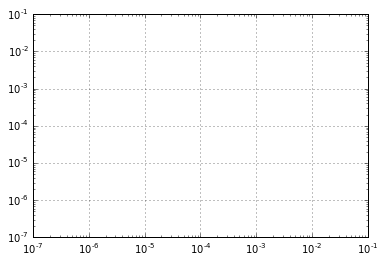We see here that each major tick shows a large tickmark and a label, while each minor tick shows a smaller tickmark with no label.

These tick properties—locations and labels—that is, can be customized by setting the formatter and locator objects of each axis. Let's examine these for the x axis of the just shown plot:

In :
print(ax.xaxis.get_major_locator())
print(ax.xaxis.get_minor_locator())

<matplotlib.ticker.LogLocator object at 0x10dbaf630>
<matplotlib.ticker.LogLocator object at 0x10dba6e80>

In :
print(ax.xaxis.get_major_formatter())
print(ax.xaxis.get_minor_formatter())

<matplotlib.ticker.LogFormatterMathtext object at 0x10db8dbe0>
<matplotlib.ticker.NullFormatter object at 0x10db9af60>


We see that both major and minor tick labels have their locations specified by a LogLocator (which makes sense for a logarithmic plot). Minor ticks, though, have their labels formatted by a NullFormatter: this says that no labels will be shown.

We'll now show a few examples of setting these locators and formatters for various plots.

## Hiding Ticks or Labels¶

Perhaps the most common tick/label formatting operation is the act of hiding ticks or labels. This can be done using plt.NullLocator() and plt.NullFormatter(), as shown here:

In :
ax = plt.axes()
ax.plot(np.random.rand(50))

ax.yaxis.set_major_locator(plt.NullLocator())
ax.xaxis.set_major_formatter(plt.NullFormatter())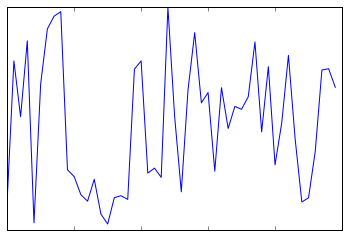Notice that we've removed the labels (but kept the ticks/gridlines) from the x axis, and removed the ticks (and thus the labels as well) from the y axis. Having no ticks at all can be useful in many situations—for example, when you want to show a grid of images. For instance, consider the following figure, which includes images of different faces, an example often used in supervised machine learning problems (see, for example, In-Depth: Support Vector Machines):

In :
fig, ax = plt.subplots(5, 5, figsize=(5, 5))

# Get some face data from scikit-learn
from sklearn.datasets import fetch_olivetti_faces
faces = fetch_olivetti_faces().images

for i in range(5):
for j in range(5):
ax[i, j].xaxis.set_major_locator(plt.NullLocator())
ax[i, j].yaxis.set_major_locator(plt.NullLocator())
ax[i, j].imshow(faces[10 * i + j], cmap="bone")Notice that each image has its own axes, and we've set the locators to null because the tick values (pixel number in this case) do not convey relevant information for this particular visualization.

## Reducing or Increasing the Number of Ticks¶

One common problem with the default settings is that smaller subplots can end up with crowded labels. We can see this in the plot grid shown here:

In :
fig, ax = plt.subplots(4, 4, sharex=True, sharey=True)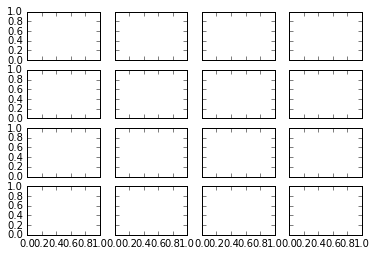Particularly for the x ticks, the numbers nearly overlap and make them quite difficult to decipher. We can fix this with the plt.MaxNLocator(), which allows us to specify the maximum number of ticks that will be displayed. Given this maximum number, Matplotlib will use internal logic to choose the particular tick locations:

In :
# For every axis, set the x and y major locator
for axi in ax.flat:
axi.xaxis.set_major_locator(plt.MaxNLocator(3))
axi.yaxis.set_major_locator(plt.MaxNLocator(3))
fig

Out: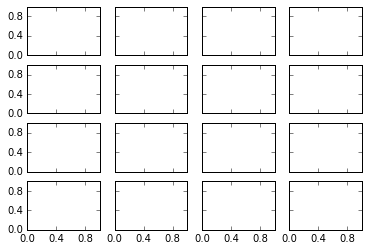This makes things much cleaner. If you want even more control over the locations of regularly-spaced ticks, you might also use plt.MultipleLocator, which we'll discuss in the following section.

## Fancy Tick Formats¶

Matplotlib's default tick formatting can leave a lot to be desired: it works well as a broad default, but sometimes you'd like do do something more. Consider this plot of a sine and a cosine:

In :
# Plot a sine and cosine curve
fig, ax = plt.subplots()
x = np.linspace(0, 3 * np.pi, 1000)
ax.plot(x, np.sin(x), lw=3, label='Sine')
ax.plot(x, np.cos(x), lw=3, label='Cosine')

# Set up grid, legend, and limits
ax.grid(True)
ax.legend(frameon=False)
ax.axis('equal')
ax.set_xlim(0, 3 * np.pi);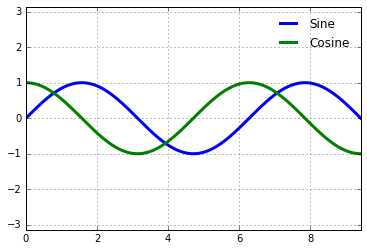There are a couple changes we might like to make. First, it's more natural for this data to space the ticks and grid lines in multiples of $\pi$. We can do this by setting a MultipleLocator, which locates ticks at a multiple of the number you provide. For good measure, we'll add both major and minor ticks in multiples of $\pi/4$:

In :
ax.xaxis.set_major_locator(plt.MultipleLocator(np.pi / 2))
ax.xaxis.set_minor_locator(plt.MultipleLocator(np.pi / 4))
fig

Out: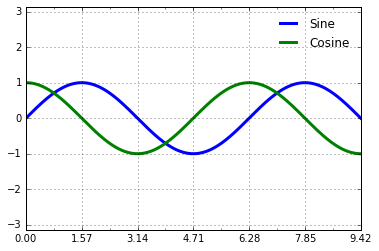But now these tick labels look a little bit silly: we can see that they are multiples of $\pi$, but the decimal representation does not immediately convey this. To fix this, we can change the tick formatter. There's no built-in formatter for what we want to do, so we'll instead use plt.FuncFormatter, which accepts a user-defined function giving fine-grained control over the tick outputs:

In :
def format_func(value, tick_number):
# find number of multiples of pi/2
N = int(np.round(2 * value / np.pi))
if N == 0:
return "0"
elif N == 1:
return r"$\pi/2$"
elif N == 2:
return r"$\pi$"
elif N % 2 > 0:
return r"${0}\pi/2$".format(N)
else:
return r"${0}\pi$".format(N // 2)

ax.xaxis.set_major_formatter(plt.FuncFormatter(format_func))
fig

Out: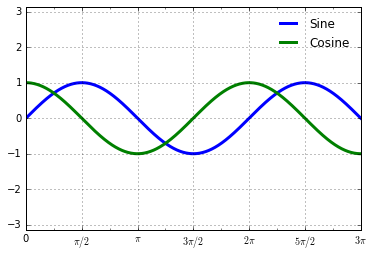This is much better! Notice that we've made use of Matplotlib's LaTeX support, specified by enclosing the string within dollar signs. This is very convenient for display of mathematical symbols and formulae: in this case, "$\pi$" is rendered as the Greek character $\pi$.

The plt.FuncFormatter() offers extremely fine-grained control over the appearance of your plot ticks, and comes in very handy when preparing plots for presentation or publication.

## Summary of Formatters and Locators¶

We've mentioned a couple of the available formatters and locators. We'll conclude this section by briefly listing all the built-in locator and formatter options. For more information on any of these, refer to the docstrings or to the Matplotlib online documentaion. Each of the following is available in the plt namespace:

Locator class Description
NullLocator No ticks
FixedLocator Tick locations are fixed
IndexLocator Locator for index plots (e.g., where x = range(len(y)))
LinearLocator Evenly spaced ticks from min to max
LogLocator Logarithmically ticks from min to max
MultipleLocator Ticks and range are a multiple of base
MaxNLocator Finds up to a max number of ticks at nice locations
AutoLocator (Default.) MaxNLocator with simple defaults.
AutoMinorLocator Locator for minor ticks
Formatter Class Description
NullFormatter No labels on the ticks
IndexFormatter Set the strings from a list of labels
FixedFormatter Set the strings manually for the labels
FuncFormatter User-defined function sets the labels
FormatStrFormatter Use a format string for each value
ScalarFormatter (Default.) Formatter for scalar values
LogFormatter Default formatter for log axes

We'll see further examples of these through the remainder of the book.